# Algebra II : Histograms

## Example Questions

### Example Question #1 : Histograms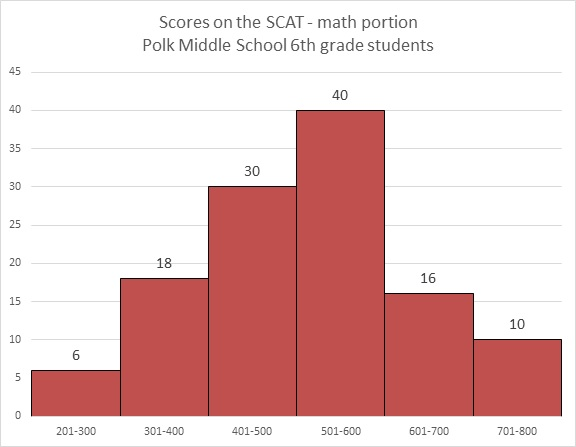Refer to the above graph. Carla, a sixth grader at Polk, outscored 101 of the students who took the test. Which of these could her score have been?

Possible Answers: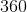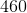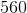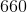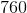Correct answer:Explanation: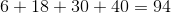students achieved scores between 200 and 600, and Carla outscored all of them.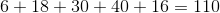students achieved scores between 200 and 700. However, Carla did not outscore all of them.

Carla's score had to have been between 600 and 700, so of the five choices, 660 is the only possible one.

### Example Question #2 : HistogramsRefer to the above bar graph.

How many students at Polk Middle School scored above 550 on the math portion of the SCAT?

Possible Answers:The answer to the question cannot be derived from the graph.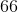Correct answer:

The answer to the question cannot be derived from the graph.

Explanation:

The dividing points of the intervals used to classify students on this graph occur at multiples of 100. No details are given for each interval beyond the number of students who scored in it, so, for example, within the interval 500-600, it is not made clear how many of the 40 students scored above or below 550. The answer is that insufficient information is provided.

### Example Question #1 : Histograms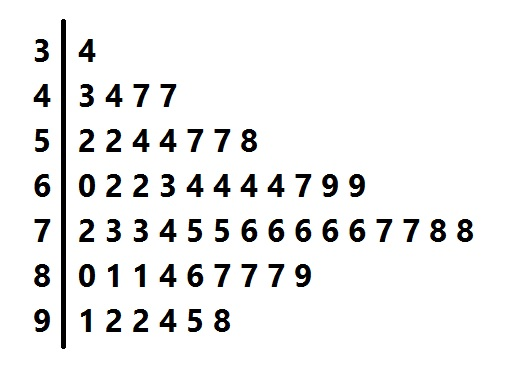Above is a stem-and-leaf representation of the scores on a test administered to a group of students. What was the midrange of the scores?

Possible Answers: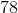Correct answer:Explanation:

The midrange is the mean of the highest and lowest scores.

Each "stem" in the left column represents the tens digits of the scores; each of the numbers in its row, or "leaf" represents the units digits. The lowest score is represented by the 4 "leaf" in the "3" row - that is, it is 34 - and the highest score is represented by the 8 "leaf" in the "9" row - that is, 98. The midrange is therefore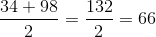### Example Question #4 : Histograms

The following histogram shows the highest level of education attained by the employees of a local store.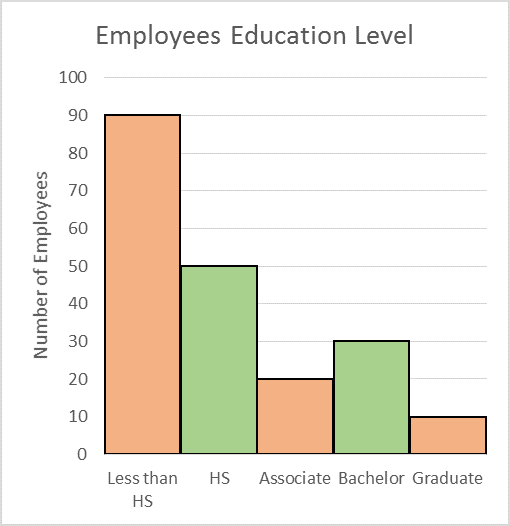How many employees are working for the store?

Possible Answers: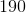Correct answer:Explanation:

Solution

The height of each bar shows the number of employees who attained that particular level of education. So to find the total number of employees, we add the numbers shown by all the bars in the histogram.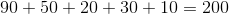The total number of employees is 200.

### Example Question #5 : Histograms

The following histogram shows the highest level of education attained by the employees of a local store.How many employees have at least an Associate degree?

Possible Answers: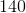Correct answer:Explanation:

Solution

The number of employees who have at least an Associate degree include the employees who have an Associate degree, the employees who have a Bachelor degree and the employees who have a Graduate degree.

We read the numbers from the 3 bars on the histogram showing the employees with Associate, Bachelor, and Graduate degrees.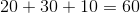### Example Question #6 : Histograms

This histogram shows the distribution of grades on Mr. Margarine's final. What percentage of the students got an A or B?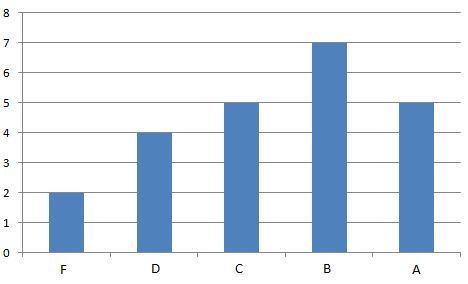Possible Answers: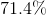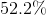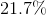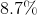Correct answer:Explanation:

The histogram shows that there are a total of 23 students.

There were 7 students who earned B's and 5 who earned A's, so that's 12 who got A's or B's.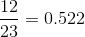, or.

### All Algebra II Resources